×

Search anything:

# Delete Middle Node from Linked ListGet this book -> Problems on Array: For Interviews and Competitive Programming

In this problem, we will delete the Middle Node from a given Linked List. We have covered the algorithm (along with implementation) to delete the middle node from a Singly Linked List and Doubly Linked List.

We will go through some of the basics of Linked List and the delete operation to remove a given node in a Linked List and then, move to our main topic. The challenge is to identify the node to delete that is the middle node in the given Linked List.

Linked List is a one of the DataStructure used for storing collection of data. A Linked List has the following properties:

1. A Linked List is linear dynamic DataStructure.
2. The number of nodes in Linked List is not fixed, it can grow and shrink on demand.
3. Each node of the Linked List is made up of two items - the data and a reference to the next node.
4. The Last node has reference to null.
5. The entry point is called head
6. If the list is empty then the head is null reference.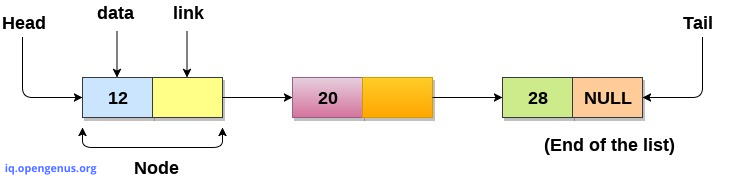# Why we use Linked List Over Array-

• The size of array must be known in advance before using it in program.
• Increasing size of the array is a time taking process. It almost impossible and to increase the size of an array at runtime.
• As array stored in our memory contiguously , inserting and deleting an element in an array means shifting of all the elements.

The Linked List consists of series of structures called Nodes. We can think of each node as a record. The first part stores the data , and second part stores a pointer to the next node. So , conclusion is each node contains two field , a data field and and next field. Generally , Linked List means Singly Linked List. In Singly Linked List , the link of the last node in the list is NULL, which indicates the end of the list.
Each Node is allocated in the heap with call to malloc() , so node memory contimues to exists until it is explicitly deallocated.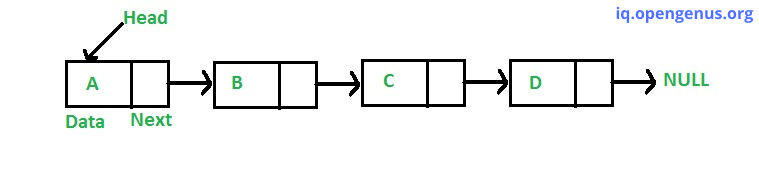The node which is called as head is the first node in the list. The last node's next pointer points to NULL value.

# Type Declaration for a Linked List of integers

``````struct ListNode
{
// define ListNode in Linked List
int data; // the data
struct ListNode *next; //pointer to the next List Node.
};
``````

# Deletion Operation on a List-

In this case, the node to be removed is always located between two nodes. Head and tails links are not updated in this case. It is done in two steps -

1. Start Traversing the Linked List, Once we find the node to be deleted , change the previous node's next pointer to the next pointer of the node to be deleted.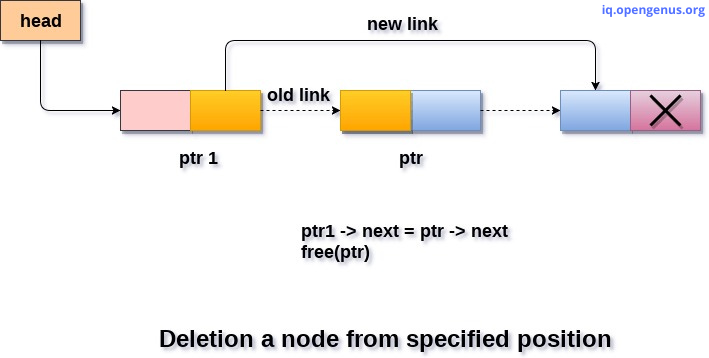1. Dispose the current node to be deleted.

# How to find the middle element?

To find the middle element, we can take two approaches:

• Uisng 2 traversals: The first traversal to find number of elements and the second traversal to find the middle element
• Using 1 traversal with slow and fast pointers

As we just need to position of middle element, we can get it using one traversal to count the total number of elements:

``````// one traversal
{
int count = 0;

while(temp.next != null)
{
++ count;
temp = temp.next;
}
return count / 2;
}
``````

# Steps to delete middle node from Singly Linked List

1. Get the position of the middle node using the above techniques (say nth position).
2. Traverse to the nth node of the singly linked list and also keep reference of n- 1 th node in some temp variable say prevnode.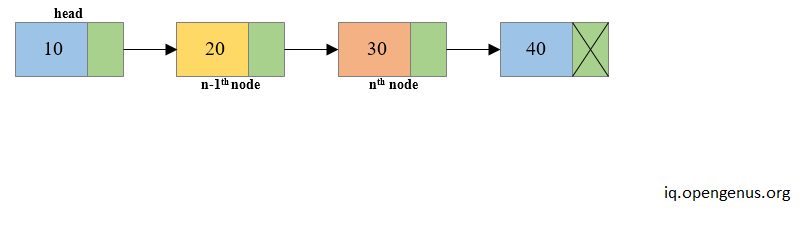1. Reconnect the n-1 th node with the n+1 th node i.e. prevNode->next = toDelete->next (Where prevNode is n-1th node and toDelete node is the nth node and toDelete->next is the n+1th node).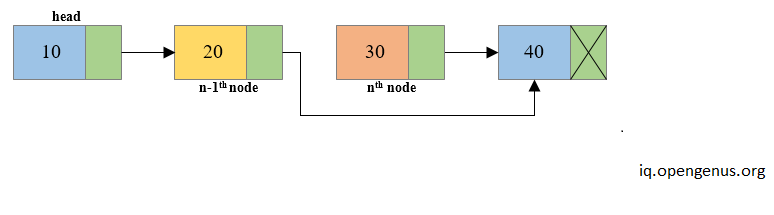1. Free the memory occupied by the n th node i.e. toDelete node.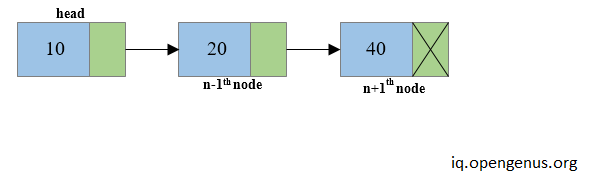# Implementation in C

``````void *delete(struct listNode **head , int position)
{
int k=1;
struct listNode *p, *q;
printf("List Empty");
return;
}

if(position == 1){ //from beginning
free(p);
return;
}

else{
//transverse the list until arriving at the position from which we want to delete
while((p!=NULL) && k<position){
k++;
q=p;
p = p->next;
}
if(p==NULL){ //at the end
printf("position doesnt exist");
return;
}
else{ //from the middle
q -> next = p ->next;
free(p);
}
return;
}
}
``````

Time Complexity - O(N) . In the worst case we may need to delete the node at the end of the list.
Space Complexity - O(1).

We can use the approach of slow and fast traversal to delete the middle node in just one traversal. The pseudocode will be like:

``````// one traversal
{

while(first_node != null && second_node != null)
{
first_node = first_node.next;
second_node = second_node.next.next;
}

delete(first_node);
}
``````

Apart from Singly Linked List , we also have two others types of Linked List i.e. Doubly Linked List and Circular Linked List.

We will look the structures of both the types and brief about how we can delete an intermediate node from these two.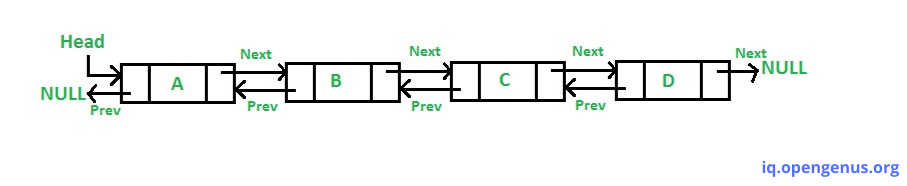A Doubly Linked List contains an extra pointer, typically called previous pointer, together with next pointer and data which are there in singly linked list.

The advantage of Doubly Linked List is that given a node in the list, we can navigate in both direction. In Doubly Linked List we can delete a node even we dont have previous node address ( since we have previous pointer ).

The disadvantage of Doubly Linked List are - Eachnmnode requires an extra pointer, requiring more space. The insertion and deletion of a node takes a bit longer.

# Deleting an intermediate Node in Doubly Linked List

In this case, the node to be removed is always located between two nodes, and heads and tails are not updated, the removal can be done in two steps-

1. Maintain the previous node while also traversing the list. Upon locating the node to be deleted , change the previous node's next pointer to the next node of the node to be deleted. Also, change the previous pointer of the next node to the node to be deleted to the point to the previous node of the node to be deleted.
2. Dispose the current Node to be deleted.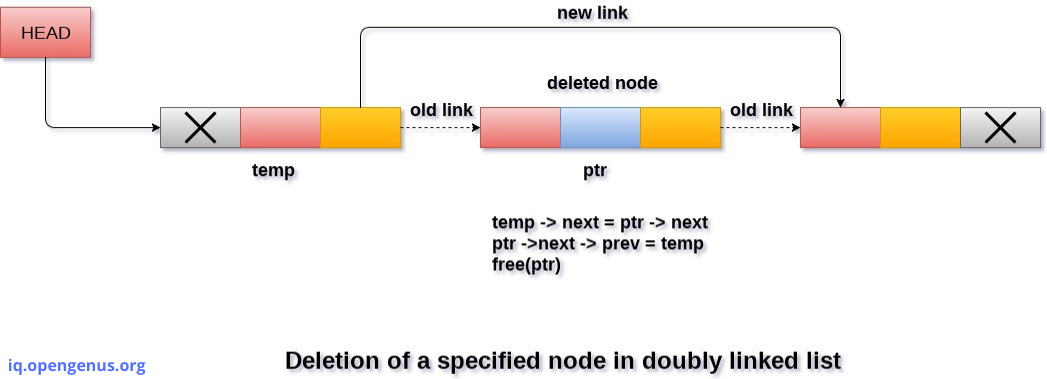Time Complexity - O(N).

Circular linked list is a linked list where all nodes are connected to form a circle. There is no NULL at the end. A circular linked list can be a singly circular linked list or doubly circular linked list.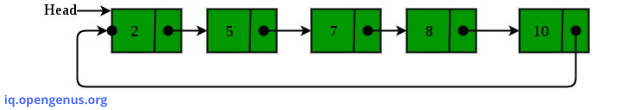1. Circular Doubly Linked Lists are used for implementation of advanced data structures like Fibonacci Heap.
2. Useful for the implementations of queues.